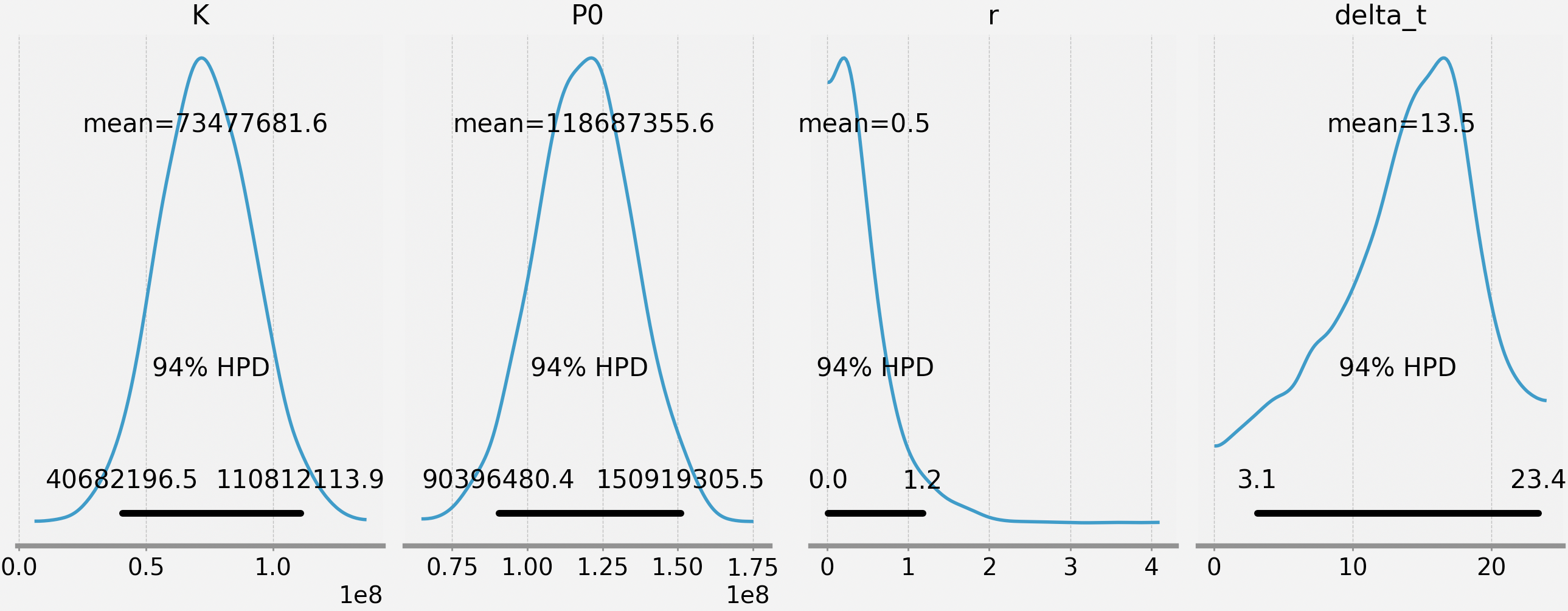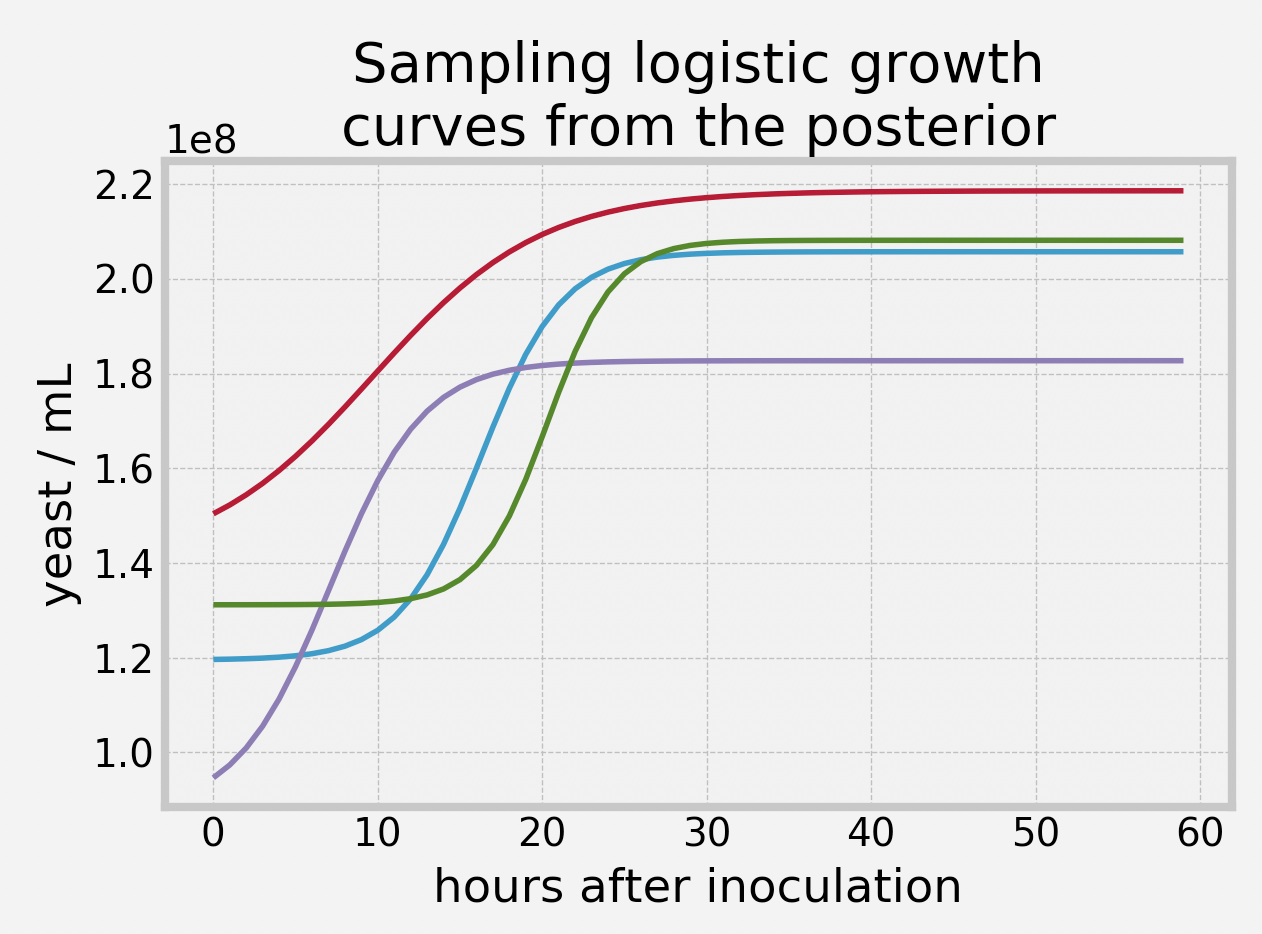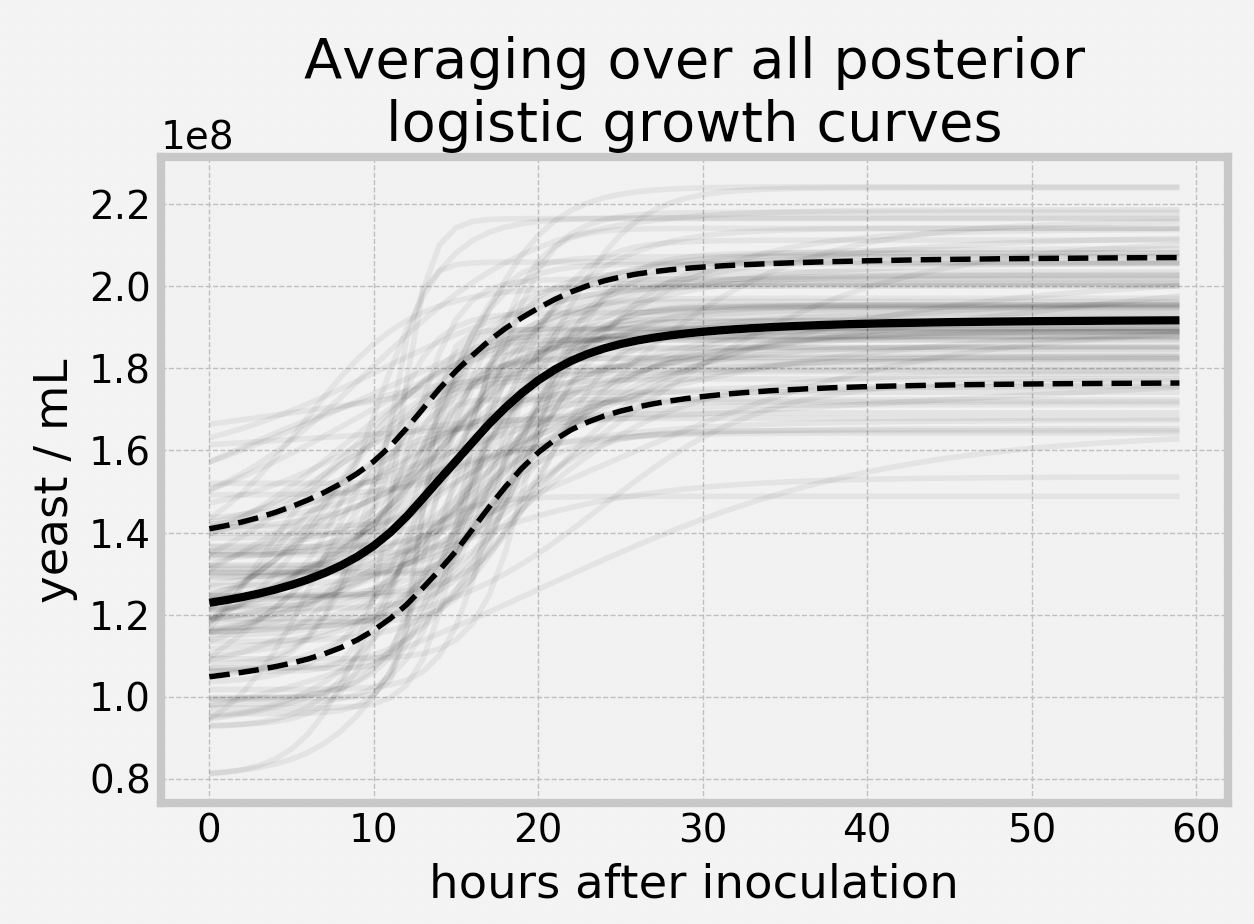# Bayesian cell counting Pt. 2 - Growth over time

Posted by Cameron Davidson-Pilon on

I’ve started growing yeast in my closet-turned-laboratory. There’s a reason why I am growing yeast, but that’ll be for another post. For this experiment, I wanted to use my new hemocytometer to do cell counts periodically over the next few days to gather data.

A nutrient-rich bioreactor (an Erlenmeyer flask with wort) was left at room temperature with plenty of aeration (a magnetic stirrer) for about 2.5 days. My collected data is below.

| hour | cell count |
|------|------------|
| 0    | 20         |
| 12.5 | 21         |
| 17.5 | 28         |
| 23   | 34         |
| 36.5 | 34         |
| 42.5 | 31         |
| 48   | 32         |
| 65   | 32         |

## Modelling

As I mentioned in my previous post, counting cells is a very noisy process, so we want to keep that in mind as we analysis this data. We have two options to proceed:

1. Assume each sample is independent, and run the same Bayesian model outlined in the previous article on each observed count, and plot the posterior distributions over time. This has the advantage of not assuming any parametric form of growth, but it has the serious disadvantage of not pooling any of the information (i.e. information at time 23 is very relevant to inference at time 17.5 and 36.5).
2. Assume some parametric growth model with unknown parameters, and fit to these parameters.

I like option two, as it’s more of a challenge, and it can be used for interpolation within the data points and extrapolation outside of the observed data points.

Typically microorganism growth after inoculation has 3 phases: lag-phase, log-phase and stationary phase. The lag-phase is a period where the organisms become accustomed to their new environment, and have low reproduction. The log-phase is a poorly-named phase that represents the period of high (exponential) reproduction. Finally, after the medium has been depleted or the organism concentration has become too high, the organisms stop reproducing and they enter the stationary phase. This type of growth looks a lot like logistic growth, so let’s use that model:

$$(\text{yeast/mL})_t = P_0 + \frac{K}{1 + \exp(-r\cdot(t - \delta))}$$

The lag-phase is modeled by the $$\delta$$ parameter - the larger this is, the longer the lag-phase went on for. From sources, the lag-phase usually lasts less than 24h. Given my initial bioreactor conditions, a lookup table suggests I should see about 50% growth. Furthermore, using the volume of the medium and the estimated concentration of my inoculate, I have an estimate for my initial concentration. All these give me priors for my estimates.

## Inference

We can model this in PyMC3 like so (this is modified code from my previous yeast-counting blog article).

import pymc3 as pm

MILLION = 1e6
TOTAL_SQUARES = 25
SQUARES_COUNTED = 4

yeast_counted =    np.array([20, 21,   28,   34, 34,   31,   32, 32])
hours_since_inoc = np.array([0,  12.5, 17.5, 23, 36.5, 42.5, 48, 65])
n_obs = yeast_counted.shape

def logistic(t, K, r, delta_t):
return K / (1 + np.exp(-r * (t - delta_t)))

with pm.Model() as model:

K = pm.Normal("K", mu=50 * MILLION, sd=25 * MILLION) # about 50% growth was expected
P0 = pm.Normal("P0", mu=100 * MILLION, sd=25 * MILLION)
r = pm.Exponential("r", lam=2.5)
delta_t = pm.Uniform("delta_t", lower=0, upper=24) # lag phase stops in the first 24 hours

yeast_conc = P0 + logistic(hours_since_inoc, K, r, delta_t)

shaker1_volume = pm.Normal("shaker1 volume (mL)", mu=9.0, sigma=0.05, shape=n_obs)
shaker2_volume = pm.Normal("shaker2 volume (mL)", mu=9.0, sigma=0.05, shape=n_obs)

yeast_slurry_volume = pm.Normal("initial yeast slurry volume (mL)", mu=1.0, sigma=0.01, shape=n_obs)
shaker1_to_shaker2_volume =    pm.Normal("shaker1 to shaker2 (mL)", mu=1.0, sigma=0.01, shape=n_obs)

dilution_shaker1 = pm.Deterministic("dilution_shaker1", yeast_slurry_volume  / (yeast_slurry_volume + shaker1_volume))
final_dilution_factor = pm.Deterministic("dilution_shaker2", dilution_shaker1 * shaker1_to_shaker2_volume / (shaker1_to_shaker2_volume + shaker2_volume))

volume_of_chamber = pm.Gamma("volume of chamber (mL)", mu=0.0001, sd=0.0001 / 20)

# why is Poisson justified? in my final shaker, I have yeast_conc * final_dilution_factor * shaker2_volume number of yeast
# I remove volume_of_chamber / shaker2_volume fraction of them, hence it's a binomial with very high count, and very low probability.
yeast_visible = pm.Poisson("cells in visible portion", mu=yeast_conc * final_dilution_factor * volume_of_chamber, shape=n_obs)

number_of_counted_cells = pm.Binomial("number of counted cells", yeast_visible, SQUARES_COUNTED/TOTAL_SQUARES, observed=yeast_counted, shape=n_obs)

trace = pm.sample(2000, tune=20000)

We are mostly interested in our posteriors for the parameters of the growth model.Actually, that’s not true: we aren’t really interested in the parameters. Most don’t have an easy interpretation (except for delta_t). What we are really interested in is the posterior of the growth curve. Recall this is a distribution. To demonstrate this, we can sample from the parameters’ posteriors and drop those values into the growth curve. For example, if we sampled 4 times:Each of these curves look very different, which should give us pause when we make inference about our growth. What happens if we keep sampling growth curves, and then average over all of them - what does that curve look like? What about the error bars on that? This is easy to do (and part of the reason I appreciate Bayesian computation). On the same graph, I’m also going to plot hundreds of potential realizations, as it’s important not to get too focused on the mean as being the “truth” - there is still lots of variation!Lovely. We can see an estimate for our initial concentration was about 122M ± 18M yeast/mL, and our final concentration was about 191M ± 15M yeast/mL. Eyeballing, the lag phase stopped just prior to 8h (though we need to reconcile this with the posterior mean of the delta_t is 13.5).

What was our estimated yield? I mentioned previously that 50% is expected - how did we do? This computation is also easy in Bayesian inference, we just look at the distribution of the ratio of yeast/mL at time 60 over yeast/mL at time 0. Doing this, the average value of this distribution is 59%.

## Conclusion

This was a fun little experiment to mix statistics and biology. Further extensions include adding covariates, and modeling death of cells too (there is a finite amount of energy in the medium, so this should happen eventually). For now, however, I’m washing the yeast and eventually going to dehydrate them.